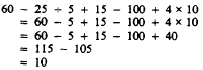multiplication may be performed in any order."> Order of OperationsCustom SearchORDER OF OPERATIONS When a series of operations involving addition, subtraction, multiplication, or division is indicated, the order in which the operations are performed is important only if division is involved or if the operations are mixed. A series of individual additions, subtractions, or multiplication may be performed in any order. Thus, In     4 + 2 + 7 + 5 = 18 or     100 - 20 - 10 - 3 = 67 or     4 x 2 x 7 x 5 = 280 the numbers may be combined in any order desired. For example, they may be grouped easily to give     6 + 12 = 18 and     97 - 30 = 67 and     40 x 7 = 280 A series of divisions should be taken in the order written. Thus,     100 ũ 10 ũ 2 = 10 ũ 2 = 5 In a series of mixed operations, perform multiplications and divisions in order from left to right, then perform additions and subtractions in order from left to right. For example     100 ũ 4 x 5 = 25 x 5 = 125 and     60 - 25 ũ 5 = 60 - 5 = 55 Now considerPractice problems. Evaluate each of the following expressions: 1.9 ũ 3 + 2  2. 18 - 2 x 5 + 4 3. 90 ũ 2 ũ 9 4. 75 ũ 5 x 3 ũ 5 5. 7 + 1 - 8 x 4 ũ 16 Answers: 1. 5           4. 9 2. 12         5. 6 3. 5 MULTIPLES AND FACTORS Any number that is exactly divisible by a given number is a MULTIPLE of the given number. For example, 24 is a multiple of 2, 3, 4, 6, 8, and 12, since it is divisible by each of these numbers. Saying that 24 is a multiple of 3, for instance, is equivalent to saying that 3 multiplied by some whole number will give 24. Any number is a multiple of itself and also of 1. Any number that is a multiple of 2 is an EVEN NUMBER. The even numbers begin with 2 and progress by 2s as follows: 2, 4, 6, 8, 10, 12, . . . Any number that Is not a multiple of 2 Is an ODD NUMBER. The odd numbers begin with 1 and progress by 2s, as follows: 1, 3, 5, 7, 9, 11, 13, . . . Any number that CM be divided into a given number without a remainder ir a FACTOR of the given number. The given number Is a multiple of any number that is one of Its factors. For example, 2, S, 4, 6; 8, and 12 are factors of 24. The following four equalities show various combinations of the factors of 24:         24 = 24 x 1         24 = 8 x 3         24 = 12 x 2        24 = 6 x 4 If the number 24 is factored as completely as possible, it assumes the form 24 = 2 x 2 x 2 x 3